Sender: Suraj Das Is there a formula for the expansion of (a+b+c) to the nth power? Does it have to do with Pascal's triangle? Student is asking. Hi Suraj, Pascal's triangle is used to find the binomial coefficients that are needed for expanding the binomial (a + b)n. The binomial coefficient n choose k is usually written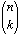or sometimes  nCk. where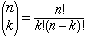In the binomial expansion of (a + b)n each term is a constant times akbn-k where the constant is n choose k. that is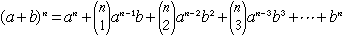(To see the first and last coefficients an binomial coefficients you need to use the conventions that 0! = 1 and x0 = 1.)    In the expansion of (a + b + c)n each term is a constant times apbqcr where p + q + r = n. In this expansion the coefficient of apbqcr is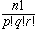. These are called multinomial coefficients. Thus (a + b + c)n is the sum of all terms of the form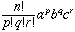where p + q + r = n. For example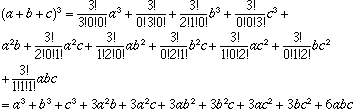Cheers, Penny Go to Math Central